Blog of Andrés Aravena
CMB2:

# Homework 6

24 March 2019. Deadline: Friday, 29 March, 9:00.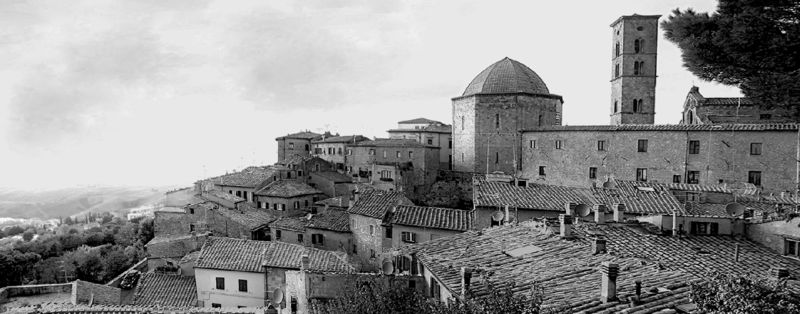Volterra is a walled town southwest of Florence, in Italy, where our imaginary story of today takes place.

# 1. The town is full of rats

The Italian town of Volterra has a big problem with rats. There are rats all around the town, running day and night, and eating all the cheese. The major of the city, Don Vito,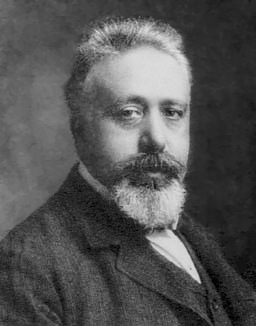Don Vito, Major of Volterra.
got some cats to deal with the problem. At first, it apparently worked, but after a few years the rats came back again and again. Every time the people think that the cats have eaten all the rats, they return and everybody complains to the major.

To understand why this happens, Don Vito has hired you as an expert on data analysis. You will work with Dr. Alfred J. Lotka,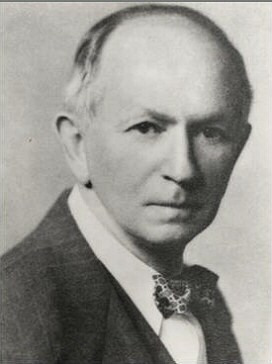Dr. Lotka, expert on cats and rats.
who will provide you with the data.

Dr. Lotka has calculated that, if there are no cats, every day the rat population increases by a birth_rate equal to 1.3%.

The rats cross through the city all day and night, running away from the cats. These cats can only survive and reproduce by catching rats. If a cat catches a rat, the rat dies and a new cat is born. Naturally, the number of rats captured (and cats born) depends on the rat population size, the cat population size, and on how good are the cats on catching rats, which we call catch_rate. Dr. Lotka thinks that catch_rate is one in one million. Rats are really scared and are running at all times. If you don’t run, you die, they say in rat tradition.

These cats are hunters. They do not eat cat food, only rats.

The cats are also running all day and night, because if they do not catch a rat, they starve and die. Dr. Lotka has measured that the death_rate, is equal 0.7% every day. If you don’t run, you die, they say in cat tradition.

On the first day of the study there are 3000 rats and 100 cats. With the data available, the Major asks you: Will the cats eat all the rats?

To figure out we will build a computational model. The “cat and rat” system can be represented by this diagram:Your mission is to write a function called cat_and_rat to simulate the cat and rat population for many days. The inputs of this function must be:

• N, the number of days that we will simulate,
• birth_rate, the birth rate for rats,
• catch_rate, the death rate of rats and birth rate of cats,
• death_rate, the death rate of cats,
• rats_ini, the initial number of rats, and
• cats_ini, the initial number of cats.

All inputs are mandatory. The output of the function must be a data frame with two columns: cats and rats.

# write your answer here

If everything is right, you can test your function with the following code that show the history of cats and rats for the next ten years:

town <- cat_and_rat(N=3650, rats_ini=3000, cats_ini=100, birth_rate=1.3/100,
catch_rate=1e-6, death_rate=0.7/100)
plot(town$cats, xlab="Days", ylab="Population", type="l") lines(town$rats, lty=2)
legend("top", legend=c("cats","rats"), lty = 1:2, inset=c(0,-0.2), horiz = TRUE, xpd = TRUE)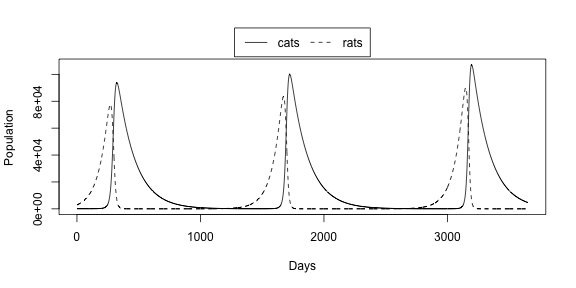We can see that at the beginning the cats catch many rats, eating almost all of them. Then everybody is happy and think that cats win and the problem is finished. But then there are so few rats that cats begin to starve and die. After near two years there are so few cats that the rats population start growing and the town is full of rodents again. So the Major can see that cats will not solve the problem.

In the output of cat_and_rat() we can find the minimum size of the rat population, given the rates and initial values. It is natural to ask how small will be the rat population if rates or initial values change. For example, you can plot min(town$rats) versus catch_rate, or depending on cats_ini. Please create a vector named initial_cats, with the sequence of numbers between 10 and 500 increasing by 10. Then calculate, for each value in initial_cats, what is the minimum number of rats in the simulation when cats_ini is a value in initial_cats. Store each result in the vector min_rats and plot it. # write your answer here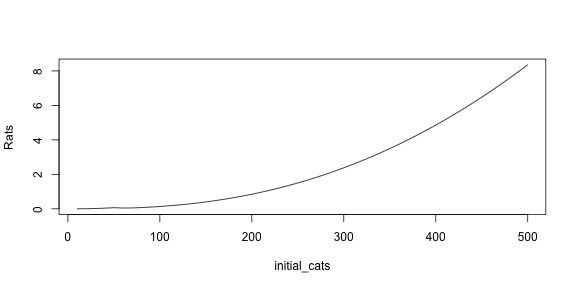## Bonus In general, you can study the effect of each rate and initial value on the rat population with different measurements, for example: • min(town$rats)
• max(town$rats) • sum(town$rats)
• locate_half(town\$rats) (as defined in homework 5)
# write your answer here

We can also see the relationship between cats and rats in the state space diagram. The state of the system is the value of all parts of the system. In this plot we can see what happens in the house in general, independent of the day.

plot(rats ~ cats, data=town)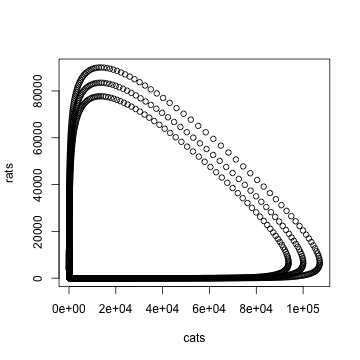We can see that, contrary to intuition, getting more cats does not reduce the minimum number of cats. On the contrary, more cats result in more surviving rats.

# 2. When the cat is away, the rats will play

Dr. Lotka thinks that these rats have some kind of genetic resistance to the cats, so we should analyze the rat genome. He wants to see if there is any atypical gene in the chromosome 20 of rat’s genome that can be useful in his research. Your mission is to identify which genes are different from the others.

Please go to the NCBI webpage of Rattus norvegicus chromosome 20, and download the Coding Sequences in FASTA format and store it on your disk. You should click on the “Send to” link and choose the appropriate options, as in the following picture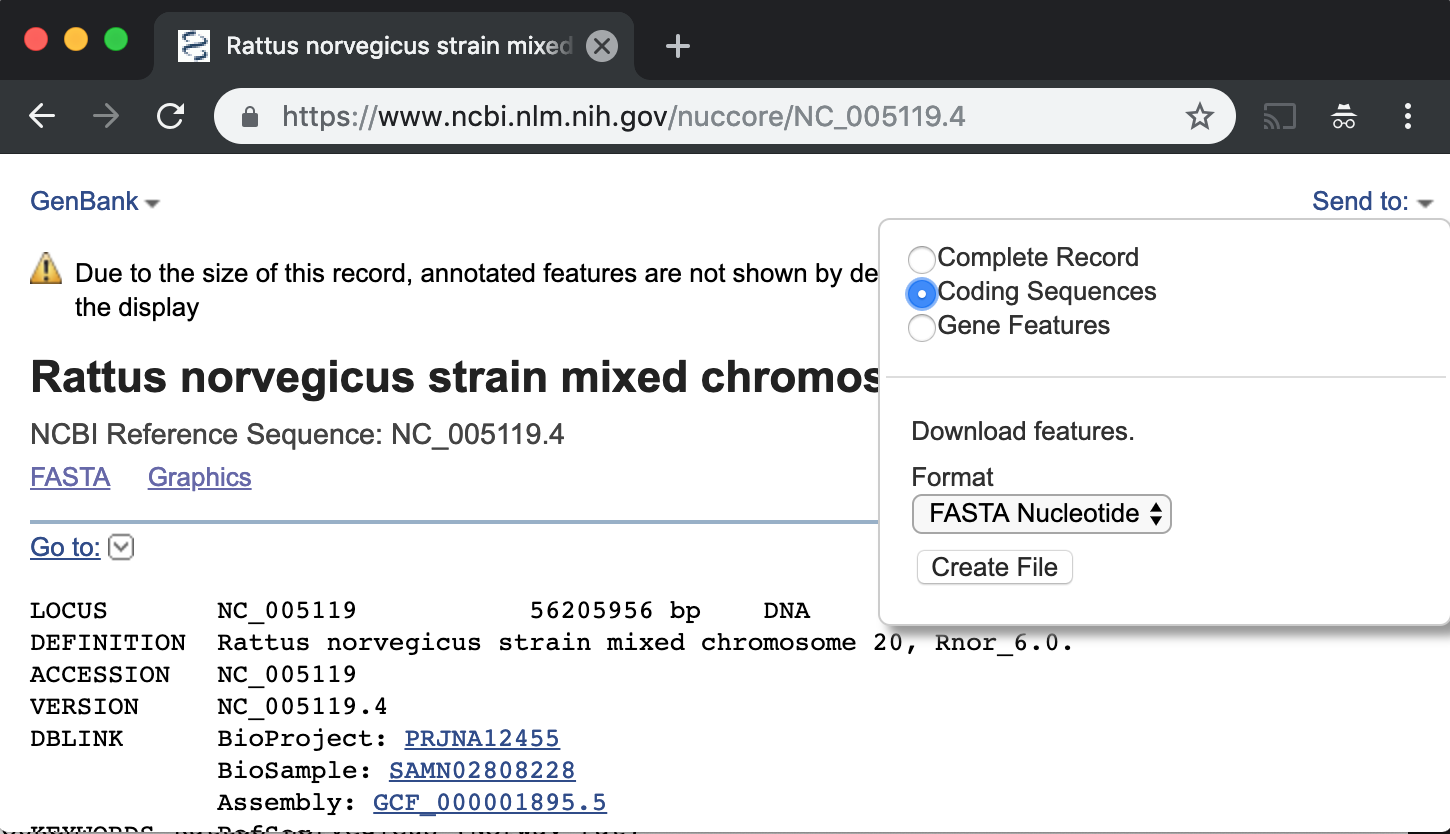This will give you a file named sequence.txt. The file is a FASTA file, even if the filename extension is .txt. Write the code to load the FASTA file into an R list called genes using the function read.fasta() from the seqinr library. Then find how many genes are in the chromosome. The result should be:

# write your answer here
 1508

## Calculate GC content

Now you have to calculate the GC content This is not the GC-skew we saw in class. The GC content is $\frac{G+C}{A+C+G+T}.$ of each gene. Fortunately the seqinr library provides a function called GC() that takes a single DNA sequence and returns the GC content. Please write the code to calculate the GC content of each gene and store it on the vector gc_genes.

# write your answer here

If everything is right you should be able to plot the following figure:

plot(gc_genes)
abline(h=mean(gc_genes))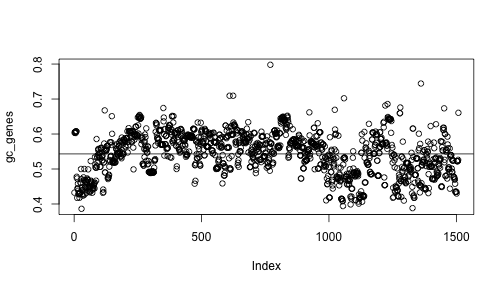## Find extreme values

Most of the genes have similar GC content. We want to know which gene has the highest GC content and which one as the lowest. Please write the code to find each one of these genes. First, find the index of maximum and of minimum in the gc_genes vector.

# write your answer here
 770

 29

Then use these indices to look inside names(genes)

# write your answer here
 "lcl|NC_005119.4_cds_XP_017457325.1_770"

 "lcl|NC_005119.4_cds_XP_017457319.1_29"

Remember that the list genes was created with the function read.fasta(). It is a named list, and the name of each element is the NCBI accession of each gene.

## Bonus

In the plot we can see that most of genes are below average in the first part of the chromosome, then above average, then below again. That is, there are three regions in the chromosome. Can you find the limits of these regions? In other words, can you find which genes are in the border of each region and draw this plot?

# write your answer here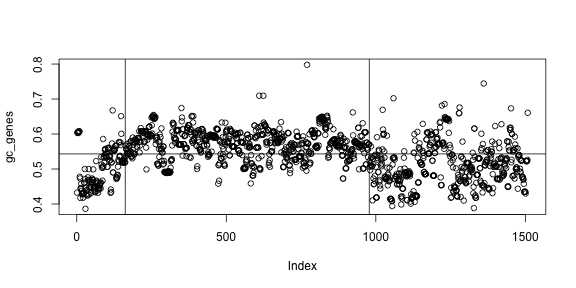# 3. A turtle draws a cat

All this cat and rat issue has been bad for tourism. To revert this situation Don Vito, the Major of the city, wants to make an art exposition to show how computers can handle cats and rats. He asks you to write a program to draw a rat like the one in the figure, using Turtle graphics.

Remember that you can move the turtle without drawing using the command turtle_setpos(x,y), and you can choose any angle using turtle_setangle(angle). For your convenience, you can also use the following functions turtle_polygon(N,D) and turtle_circle(D) defined here:

turtle_polygon <- function(N, D) {
side <- D * sinpi(1/N)
turtle_do({
turtle_left(90-180/N)
for(x in 1:N) {
turtle_forward(side)
turtle_right(360/N)
}
})
}

turtle_circle <- function(D) {
turtle_polygon(180, D)
}

In both functions the parameter D indicates the diameter of the polygon or circle. When you use turtle_polygon() the parameter N indicates the number of sides on the polygon. For example turtle_polygon(3) draws a triangle. The center of the polygon is always in the direction where the turtle was looking.

The rat’s body has diameter 36, the head’s diameter is 20 and the ears have diameter 10. The eyes have diameter 4 and the nose size is 2.5.

# write your answer here

## Bonus

Write also the code to draw the cat.

# write your answer here

# Colophon

This story is fictional but the analysis is real. If you want to know the real story, ask Google about “Lotka Volterra”.

Remember: The more exercises you do, the more chances you have to pass the course.